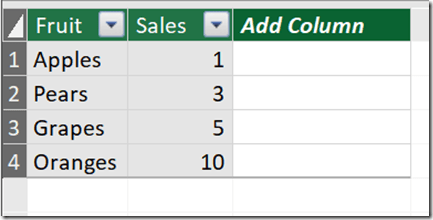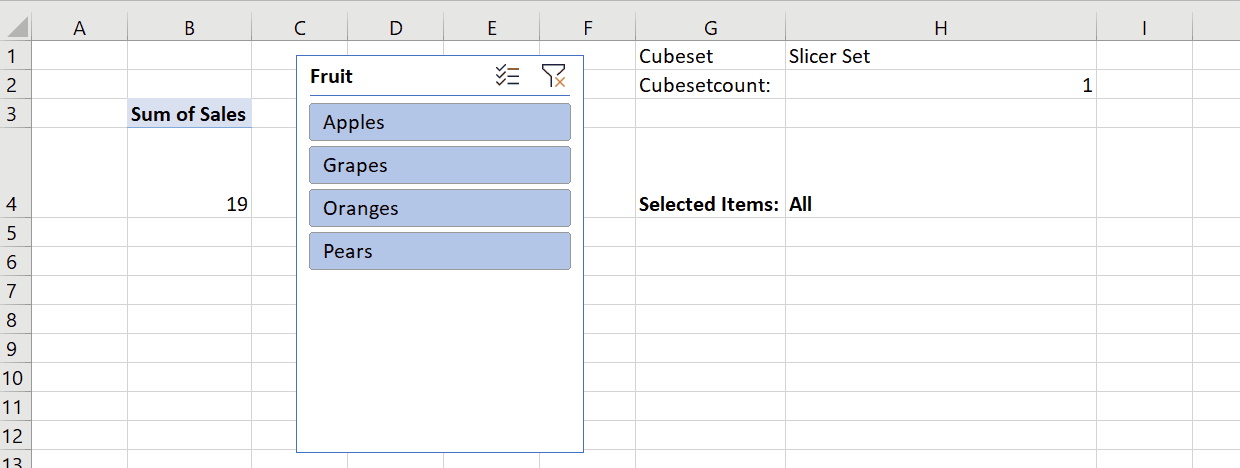# Finding All Selected Items In An Excel Slicer Connected To SSAS, Power BI Or the Excel Data Model Using Dynamic Arrays

The big news in the world of Excel right now is the introduction of dynamic arrays. They’re only available in the Office 365 click-to-run version of Excel and, at the time of writing, only available to people on the Office Insiders programme, but eventually they’ll be available to anyone running Excel for Office 365 on their desktop. There are already lots of blog posts about them including this overview by Jon Acampora, and you probably also want to download Bill Jelen’s detailed mini-book on them here which is free for the rest of 2018. Now I’m not an Excel expert by any stretch of the imagination but I’m as excited as anyone else about them because they will be incredibly useful for anyone building reports using Excel cube functions against Analysis Services, the Excel Data Model/Power Pivot and Power BI. Bill Jelen’s book has a short section on this subject but the possibilities are limitless…

Here’s one example of how they can be used. A while ago I blogged about how to use a regular array formula and the TextJoin() Excel function to get all the selected items from a slicer. Dynamic arrays make this problem much easier to solve. Take the following table loaded into the Excel Data Model:Now, say you have a PivotTable built from this and a slicer (called Slicer_Fruit) connected to it:It’s possible to use the CubeSet() function to get the set of selected items in a slicer using the following formula:

```=CUBESET("ThisWorkbookDataModel",Slicer_Fruit,"Slicer Set")
```

Assuming this formula is in cell H1, you can then get the number of items in this set using CubeSetCount():

```=CUBESETCOUNT(\$H\$1)
```

Assuming this is in cell H2, all you need to do to get a comma-delimited list of all the selected items in the slicer via this set is:

```=
TEXTJOIN(
", ",
TRUE,
CUBERANKEDMEMBER(
"ThisWorkbookDataModel",
\$H\$1,
SEQUENCE(\$H\$2,1)
)
)
```Here it is in action:It works as follows:

• The new Sequence() function is used to create a dynamic array of numbers from one to the number returned by the CubeSetCount() function.
• The CubeRankedMember() gets the name of each of the items in the set using the numbers returned by Sequence()
• TextJoin() then concatenates all of the names returned by CubeRankedMember() into a single comma-delimited list.

You can download the sample workbook here (remember it will only work if you have a version of Excel installed that includes dynamic arrays!).

### 7 responses

1.Olivier Travers (@otravers) says:

Very nice! The last time I tried to do this with cube formulas, I wasted hours to no avail.

2.sam says:

@Chris
Here are some interesting ways of using Dynamic Array Formulas

Sample File

https://1drv.ms/f/s!AiKBTsYfZw-vgrtkdDXnLzxgimxokg

1. Multi Criteria SUMIFS / COUNTIFS

=SUMIFS(D[COST],D[CLIENT],INDEX(N2#,,1),D[REGION],INDEX(N2#,,2))

2. Multi Column Sort

=SORT(D,{1,2,3,4},{1,1,1,-1})

3. Sort by Custom List e.g North, South, East, West or Jan, Feb, Mar..

=SORTBY(D,D[CLIENT],1,MATCH(D[REGION],CustList,0),1,D[COST],-1)

CustList is either a named Range containing North, South, East, West or an array Constant

4. Emulate Wild cards in the Filter Function

a) Contains (*Text*)

=FILTER(D,IFERROR(SEARCH(K1,D[CLIENT]),0),”No Data”)

b) Begins with (Text*)

=FILTER(D,IFERROR(LEFT(D[CLIENT],LEN(Q1))=Q1,0),”No Data”)

c) Ends with (*Text)

=FILTER(D,IFERROR(RIGHT(D[CLIENT],LEN(V1))=V1,0),”No Data”)

5. Clients with Characters (=???)

=FILTER(D,IFERROR(LEN(D[CLIENT])=LEN(AC1),0),”No Data”)

6. Extract a user defined number of Random Records from a Dataset

Where RandIndex = NDEX(SORTBY(SEQUENCE(ROWS(D)),RANDARRAY(COUNTA(SEQUENCE(ROWS(D))))),SEQUENCE(NoOfSamples))

7. Multiple OR Criteria

a) Clients : ABB or BHEL or ORACLE

=FILTER(F,COUNTIFS(mClients,F[CLIENT]))

b) BU : US or DE or GB

=FILTER(F,MMULT(IFERROR(–(SEARCH(TRANSPOSE(mBU),F[BU])=1),0),SEQUENCE(COUNTA(mBU),,,0)))

c) Cost (> 10 and 90 and 10)*(F[COST]90)*(F[COST]<100))),5)

8. Remove and Rearrange Columns

=INDEX(F,SEQUENCE(ROWS(F)),{3,5,1})

Enjoy

Sam

3.Oleksiy says:

This is also possible w/o SEQUENCE function:
= TEXTJOIN(
“, “,
TRUE,
CUBERANKEDMEMBER(
“ThisWorkbookDataModel”,
Slicer_Fruit,
ROW(INDEX(OFFSET(A1,0,0,CUBESETCOUNT(Slicer_Fruit)),,1))
)
)

4.Oleksiy says:

@Sam – were you a student at Excel Hero Academy?

This site uses Akismet to reduce spam. Learn how your comment data is processed.